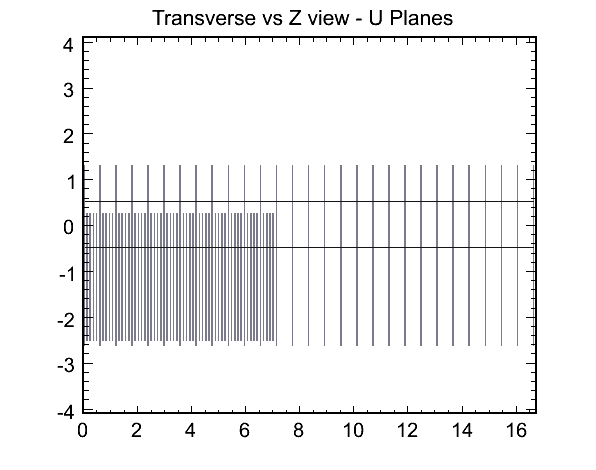# Grey-scale, color scale coexistance?

Hi,

I’d like to draw a few 2d histograms in gray-scale, but draw others with the usual pretty palette. I know how to construct the gray-scale:

``````int ncol=10;
double red[ncol];
double green[ncol];
double blue[ncol];
double stops[ncol];

double dcol = 1/double(ncol);
double gray = 0;
for (int j = 0; j < ncol; j++) {
//   ...... Define color with RGB equal to : gray, gray, gray .......
stops[j]=double(j)/double(ncol-1);
red[j]=gray;
blue[j]=gray;
green[j]=gray;

gray += dcol;
}
double totcol=50;```{ TCanvas *c1 = new TCanvas("c1"); TH2F *h1 = new TH2F("h1","h1",40,-4,4,40,-4,4); TH2F *h2 = new TH2F("h2","h2",40,-4,4,40,-4,4); Double_t a,b; for (Int_t i=0;i<5000;i++) { gRandom->Rannor(a,b); h1->Fill(a-1.5,b-1.5); h2->Fill(a+1.5,b+1.5); } TExec *ex1 = new TExec("ex1","gStyle->SetPalette(0);"); TExec *ex2 = new TExec("ex2","gStyle->SetPalette(1);"); h1->Draw("colz"); ex1->Draw(); h1->Draw("col same"); ex2->Draw(); h2->Draw("col same"); }```